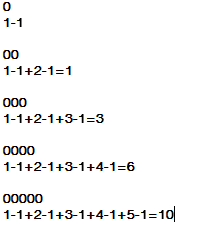PLMU - Editorial

Author: Vivek Chauhan
Tester: Ildar Gainullin
Editorialist: Oleksandr Kulkov

DIFFICULTY:
CAKEWALK
PREREQUISITES:
None
PROBLEM:
You’re given a sequence A_1, \dots, A_n. Find the number of pairs (i,j) such that A_i + A_j = A_i \cdot A_j.
QUICK EXPLANATION:
Keep an eye on twos and zeros.
EXPLANATION:
We may rewrite it as A_i=A_j(A_i-1), or A_j = \frac{A_i}{A_i-1}. Since A_j is integer it’s only possible when either A_i=A_j=2 or A_i=A_j=0, so we just have to calculate amount of occurences of 0's and 2's in the sequence.

void solve() {
int n;
cin >> n;
map<int, int> cnt;
for(int i = 0; i < n; i++) {
int ai;
cin >> ai;
cnt[ai]++;
}
cout << cnt * (cnt - 1) / 2 + cnt * (cnt - 1) / 2 << endl;
}

AUTHOR’S AND TESTER’S SOLUTIONS:
Tester’s solution can be found here.
Editorialist’s solution can be found here.

1 Like

@vivek_1998299 , can you please explain why we use n*(n-1)/2

1 Like

nC2 = n*(n-1)/2 , is the number of ways to select 2 objects from n objects.

4 LikesI just found this pattern and derived that formula.

1 Like

can you please explain the derivation…@manjot1151

formula of sum of first n natural number !! trying to find all possibilities !!

The positives are 1+2+3+...n and negatives are -1-1-1... n times. So you can say its the sum of all positives from 1 to n and n*-1 or -n. There is a formula for sum of integers from 1 to n that is n*(n+1)/2 So now if you subtract n from it. It will become
n*(n-1)/2 and you have to do it for both 0 and 2 and then add both

@suyashs52
\LARGE^{n}\textrm{C}_{2} = \frac{n!}{2!(n-2)!} = \frac{n(n-1){\color{Red} (n-2)!}}{2{\color{Red} (n-2)!}} = \frac{n(n-1)}{2}

1 Like

This worked for you?
i did same in my code it was giving SIGFPE
pair+=(fact(c0)/(2*fact(c0-2))); // c0 is the count of number of zeros

consider the case where c0=0 means no zero present then in denominator fact(c0-2) becomes fact(-2) . same error will occur for c0 =1 .Have you handled these cases ?
Just guess the error . If it is not the reason then post you code or submission link.

1 Like

https://www.codechef.com/viewsolution/28192830
sorry for the delay

here in this question value of N≤40,000. Now assume that all are zero.In that senario is int capable of storing 400000! ? No lt can’t.Even long long is not capable of storing that huge value. So use the formula nC2 = n*(n-1)/2. In line no 19 change data type of ans[t] from int to long long and update the line no 42 to 50 as follows

long long pair=0;
if(c0>1)
{
pair+=(long long) (c0*(c0-1))/2;
}
if(c1>1)
{
pair+=(long long) (c1*(c1-1))/2;
}

N.B-You can also change the data type of c0,c1 as long long in that case you don’t need the type casting.

2 Likes

what is wrong in this code. I am getting WA.
#include
#include<string.h>
using namespace std;
int main(){
int t,n;
cin>>t;
for(int i=0; i<n; i++){
cin>>n;
int arr[n];
int two=0, zero=0,coun=0,j;
for(j=0; j<n; j++){
cin>>arr[j];
if(arr[j]==2){
two++;
//cout<<two<<endl;
}
if(arr[j]==0){
zero++;
//cout<<zero<<endl;
}
}
//cout<<two<<" “<<zero;
coun=two*(two-1)/2 + zero*(zero-1)/2;
cout<<coun<<”\n";
}
}

#include <bits/stdc++.h>
using namespace std;
#define fastIO ios_base::sync_with_stdio(0); cin.tie(0);
typedef long long int ll;
bool checkProdSum(ll x,ll y)
{
return(((y==x&&y==2)||(y==x&&y==0))?true:false);
}
int main() {
fastIO;
ll T;
cin>>T;
vector<ll>A;

while(T--)
{
ll N,input,count=0;
cin>>N;
for(int i=0;i<N;i++)
{
cin>>input;
A.push_back(input);
}
for(int i=0;i<N;i++)
{
for(int j=i+1;j<N;j++)
{
if(A[j]!=1)
{
if(checkProdSum(A[i],A[j]))
{
// cout<<i<<" "<<j<<endl;
++count;}
}
}
}
cout<<count<<endl;
A.clear();
}

return 0;
}

This code is not passing the 2nd sub-task while gives AC in the first!

1 Like

There are only 2 cases to consider and we can consider them separately. If there is 0 and 0 and if there is 2 and 2. We can have a counter for the number of 0’s and the number of 2’s. Then we add up all the numbers below the # of 0’s and the # of 2’s. Code is below:

#include <bits/stdc++.h>
using namespace std;

#define ll long long
#define pb push_back

int n;

int count(int a) {
ll sum = 0;
for(int i = 0; i <= a; i++) {
sum += i;
}
return sum;
}

void solve() {
cin >> n;
int a[n];
for(int i = 0; i < n; i++) {
cin >> a[i];
}
sort(a, a + n);
int cnt = 0;
int counter = 0;
for(int i = 0; i < n; i++) {
if(a[i] == 2) {
cnt++;
}
if(a[i] == 0) {
counter++;
}
}
cout << count(cnt - 1) + count(counter - 1) << "\n";
}

int main() {
ios::sync_with_stdio(0);
cin.tie(0);

int t;
cin >> t;
while(t--) {
solve();
}
}

On the 7th line of your code, you have

for(int i=0; i<n; i++)

the variable n holds garbage right now because you never did

cin >> n;

Also you might consider doing

for(int i=0; i<t; i++)

because t is the testcases.

Bcz
Ai + Aj = AI.Aj
Ai = Ai.Aj - Aj
Ai = Aj(Ai - 1)

Hope u get it

But why are we doing this, the question is asking for the number of pairs not for the number of ways we can choose a pair.